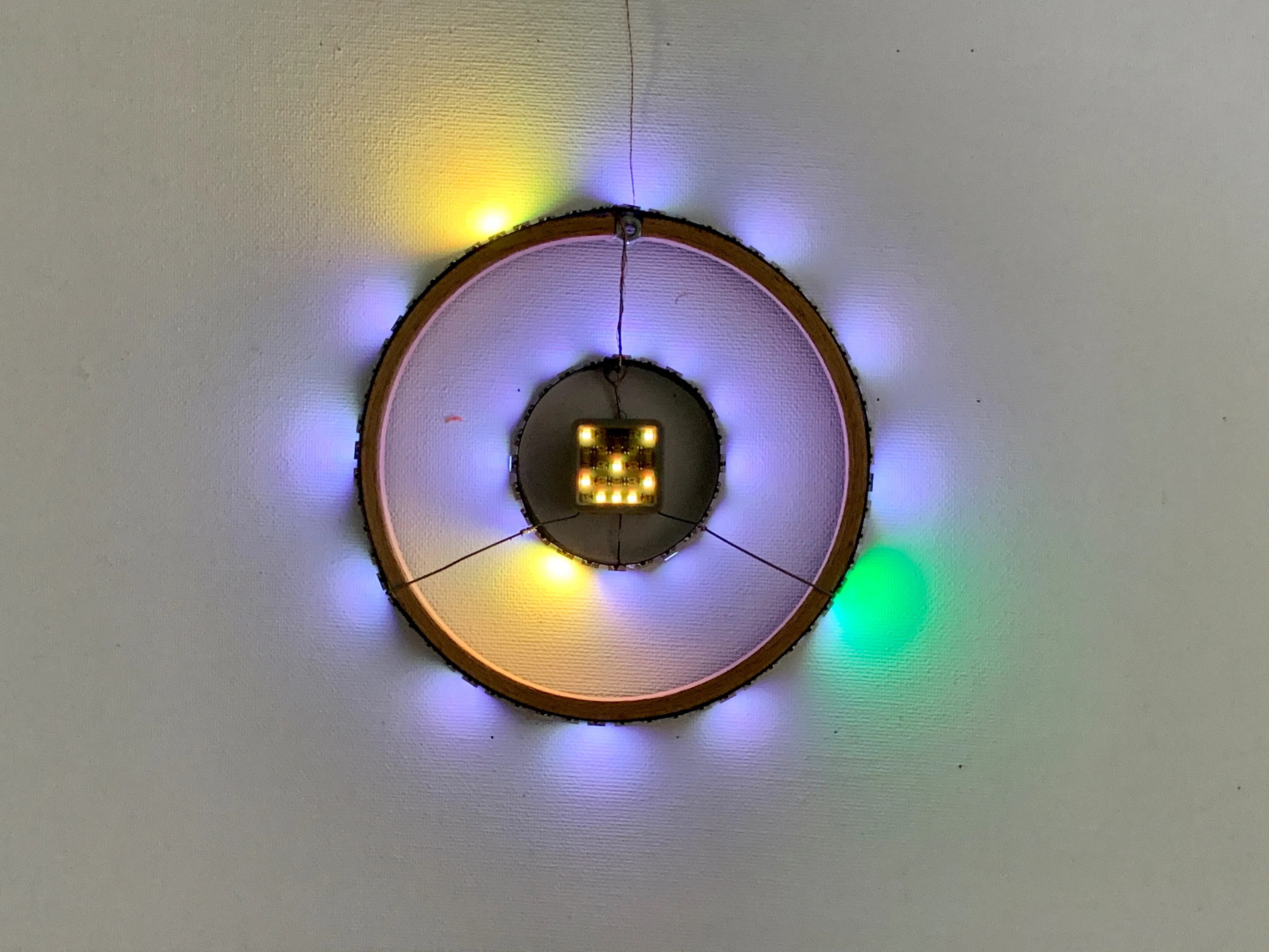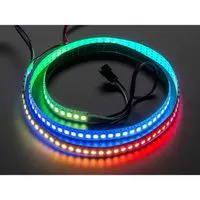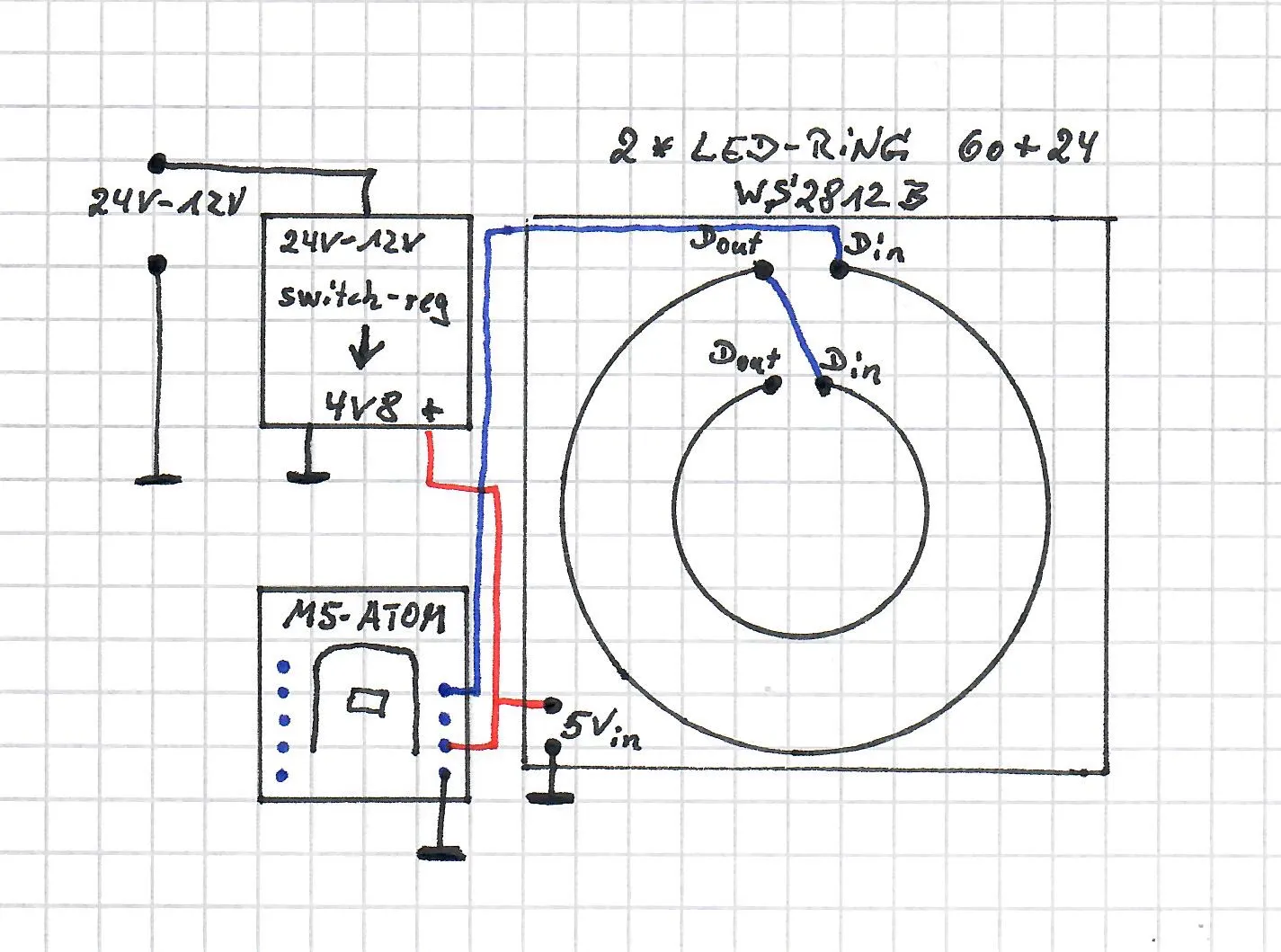Published

# ATOM Wall Clock

Wall clock shows time on two concentric NeoPixel LED rings. The inner ring shows the hours, the outer ring shows minutes and seconds## Things used in this project

### Hardware components

 M5Stack ATOM MATRIX or lite
×1Adafruit NeoPixel Digital RGB LED Strip 144 LED, 1m White
×1
 switch regulator, step down 12V-24V to 4V8
×1

## Schematics

### schematic of ATOM Wall Clock

The simple schematic of the ATOM Wall Clock## Code

### Micropython code for ATOM Wall Clock

MicroPython
Code for the ATOM Wall Clock
```import time
from machine import Pin
from neopixel import NeoPixel
#WLAN credentials
SSID   = 'WLAN1_2.4GHz'
PASSWD = 'xyz'
#color for second-hand
RS=0
GS=20
BS=0
#color for minute-hand
RM=60
GM=50
BM=0
#color for hour-hand
RH=60
GH=50
BH=0
#color for background
RB=0
GB=0
BB=0
#color for markers
Rmark=6
Gmark=6
Bmark=6

BUTTON = Pin(39,Pin.IN)

#27= internal Neopixel
# seta a single Pixel on ATOM
# and a smily on ATOM-Matrix
pin = Pin(27,Pin.OUT)
np = NeoPixel(pin, 25)
for i in (0,4,12,15,19,21,22,23):
np[i] = (int(RM/2),int(GM/2),int(BM/2))
np.write()
#time.sleep(2)

#26 = groveport pin3
#pin = Pin(26,Pin.OUT)

#21  = ATOM-Rueckseite
pin = Pin(21,Pin.OUT)

np = NeoPixel(pin, 85)
for i in range(0,83):
np[i] = (85-i,i-80,1)
np.write()

import network
wlan = network.WLAN(network.STA_IF)
wlan.active(True)
wlan.connect(SSID, PASSWD)
while not wlan.isconnected():
time.sleep(1)
print('WLAN:', wlan.ifconfig())

from machine import RTC
rtc = RTC()
t_old = rtc.datetime()
#rtc.datetime((2017, 8, 23, 1, 12, 48, 0, 0)) # set a specific date and time
import ntptime
ntptime.settime() # set the rtc datetime from the remote server

def my_show_neo(t):
##########################
# correct hh for TZ Berlin, but no DST possible
hh = ( t+2 ) % 24
mm = t
ss = t
print(hh,":",mm,":",ss," BUTTON:",BUTTON.value())
#   Clear all NeoPixel
for i in range(0,84):
np[i] = (RB,GB,BB)
#   Marker for hours on outer ring
for i in range(0,60,5):
if (i % 15 == 0):
np[i] = (Rmark,Gmark,Bmark)
else:
np[i] = (int(Rmark/2),int(Gmark/2),int(Bmark/2))
#   Marker for hours on inner ring
for i in range(60,85,2):
if (i % 6 == 0):
np[i] = (Rmark,Gmark,Bmark)
else:
np[i] = (int(Rmark/2),int(Gmark/2),int(Bmark/2))
#   set actual hour-LED on inner ring (in three steps depending on minutes)
if mm > 40:
hhh = 2
elif mm > 20:
hhh = 1
else:
hhh = 0
np[((hh % 12) *2) + 60 + hhh] = (RH,GH,BH)
#   set actual minute on outer ring
np[mm] = (RH,GH,BH)
#   set second on outer ring
np[ss] = (RS,GS,BS)
#   Now show the new pixel pattern
np.write()

return
#################################################

print('Current date and timne: ',rtc.datetime())    # get the date and time in UTC
# MAIN_LOOP
TOGGLED_by_BUTTON = 1
xs=0
xm=10
xh=8
while True:
if not BUTTON.value():
TOGGLED_by_BUTTON = 1-TOGGLED_by_BUTTON
time.sleep(2)

if TOGGLED_by_BUTTON:
# --------------------- normalCLOCK-MODUS------------
t  = rtc.datetime()
if t == t_old:
time.sleep(0.1)
else:
my_show_neo(t)
t_old = t
else:
# ---- DEMO-MODUS with fast running minutes and hours
xs += 60
if (xs >59):
xs = 0
xm += 1
if (xm >59):
xm = 0
xh +=1
if (xh >24):
xh =0
t = (0,0,0,0,xh,xm,xs)
my_show_neo(t)
print ("DEMO:",t)

#END
###########################################################
```

## Credits

### Peter Neufeld

4 projects • 5 followers